Скачать презентацию On the Cost and Benefits of Procrastination Approximation

• Количество слайдов: 37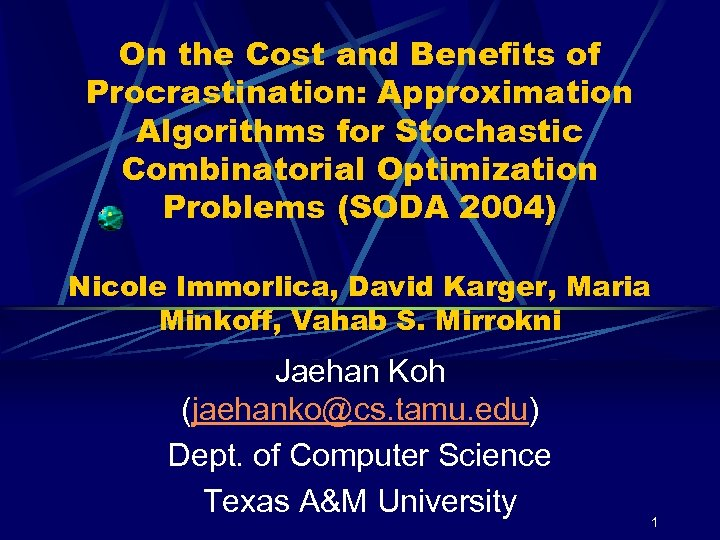On the Cost and Benefits of Procrastination: Approximation Algorithms for Stochastic Combinatorial Optimization Problems (SODA 2004) Nicole Immorlica, David Karger, Maria Minkoff, Vahab S. Mirrokni Jaehan Koh ([email protected] tamu. edu) Dept. of Computer Science Texas A&M University 1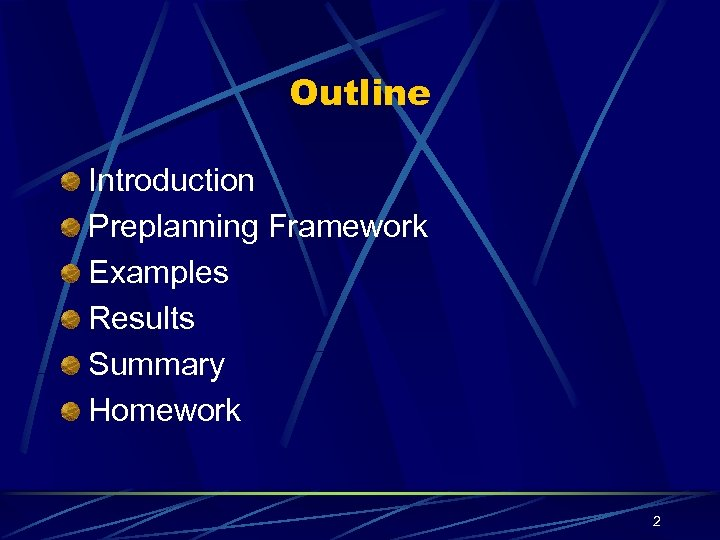Outline Introduction Preplanning Framework Examples Results Summary Homework 2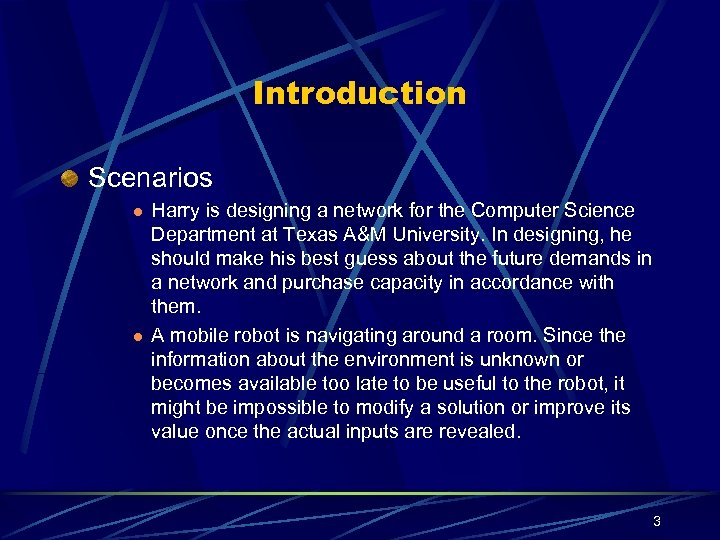Introduction Scenarios l l Harry is designing a network for the Computer Science Department at Texas A&M University. In designing, he should make his best guess about the future demands in a network and purchase capacity in accordance with them. A mobile robot is navigating around a room. Since the information about the environment is unknown or becomes available too late to be useful to the robot, it might be impossible to modify a solution or improve its value once the actual inputs are revealed. 3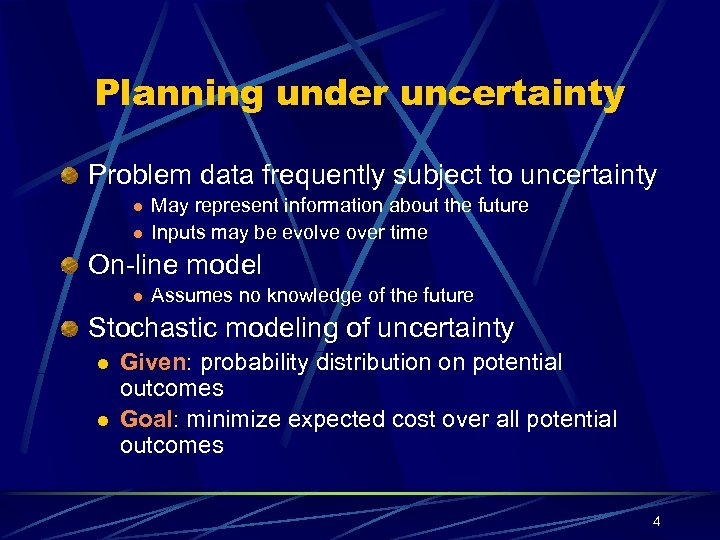Planning under uncertainty Problem data frequently subject to uncertainty l l May represent information about the future Inputs may be evolve over time On-line model l Assumes no knowledge of the future Stochastic modeling of uncertainty l l Given: probability distribution on potential outcomes Goal: minimize expected cost over all potential outcomes 4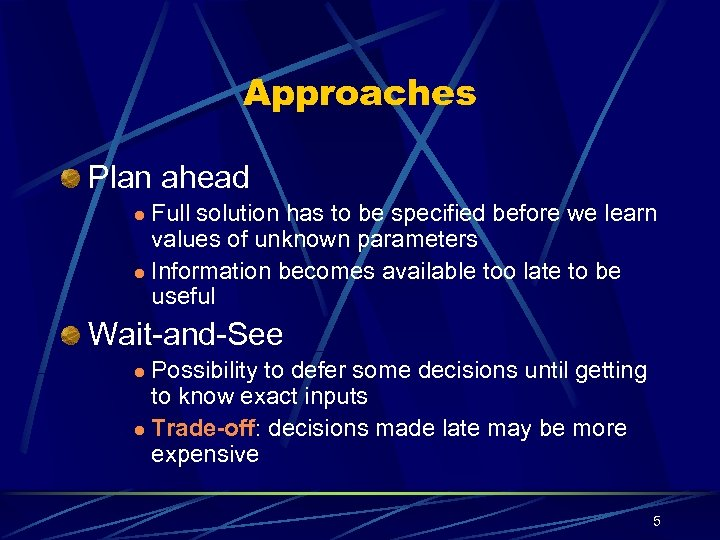Approaches Plan ahead Full solution has to be specified before we learn values of unknown parameters l Information becomes available too late to be useful l Wait-and-See Possibility to defer some decisions until getting to know exact inputs l Trade-off: decisions made late may be more expensive l 5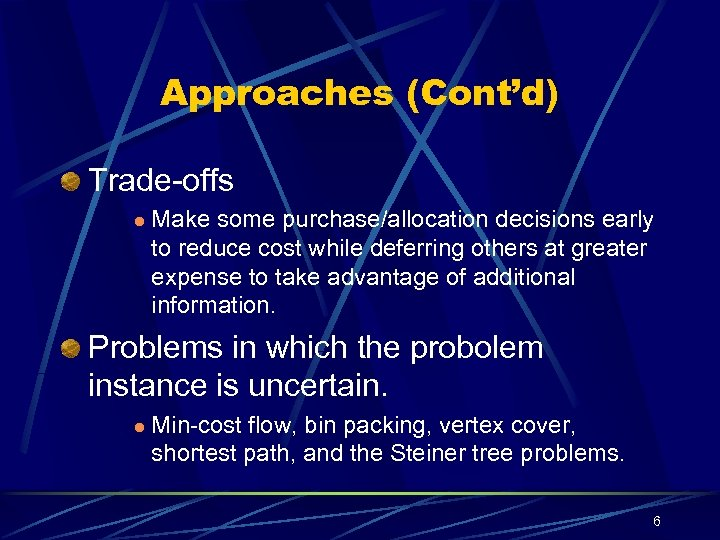Approaches (Cont’d) Trade-offs l Make some purchase/allocation decisions early to reduce cost while deferring others at greater expense to take advantage of additional information. Problems in which the probolem instance is uncertain. l Min-cost flow, bin packing, vertex cover, shortest path, and the Steiner tree problems. 6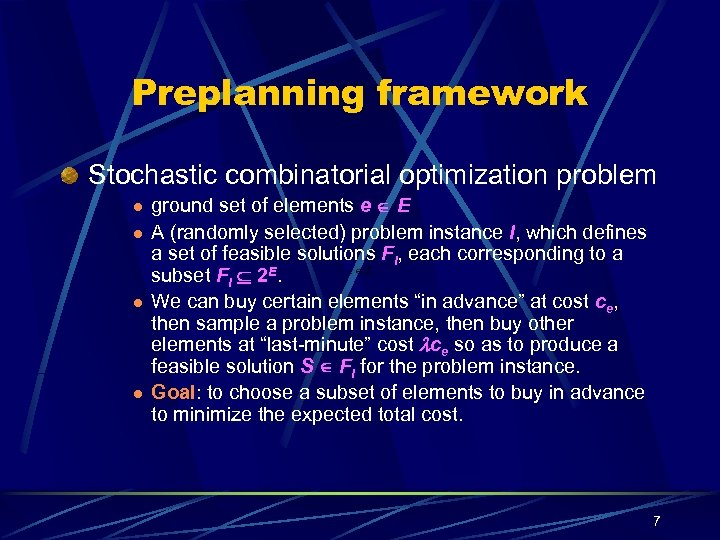Preplanning framework Stochastic combinatorial optimization problem l l ground set of elements e E A (randomly selected) problem instance I, which defines a set of feasible solutions FI, each corresponding to a subset FI 2 E. We can buy certain elements “in advance” at cost ce, then sample a problem instance, then buy other elements at “last-minute” cost ce so as to produce a feasible solution S FI for the problem instance. Goal: to choose a subset of elements to buy in advance to minimize the expected total cost. 7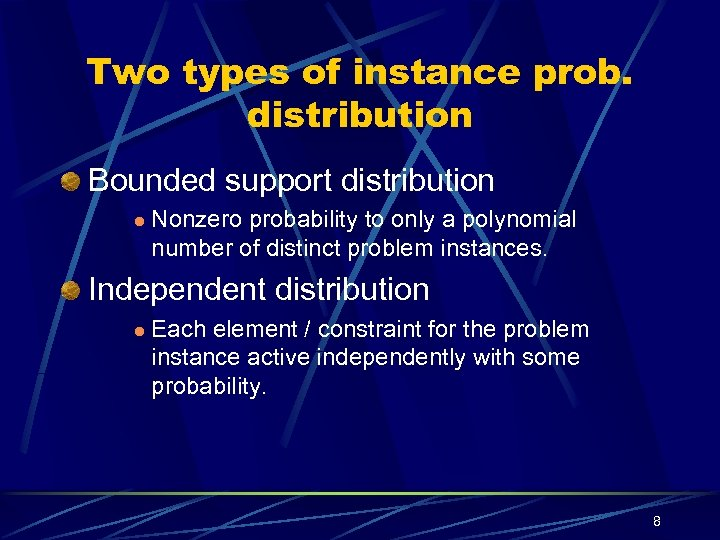Two types of instance prob. distribution Bounded support distribution l Nonzero probability to only a polynomial number of distinct problem instances. Independent distribution l Each element / constraint for the problem instance active independently with some probability. 8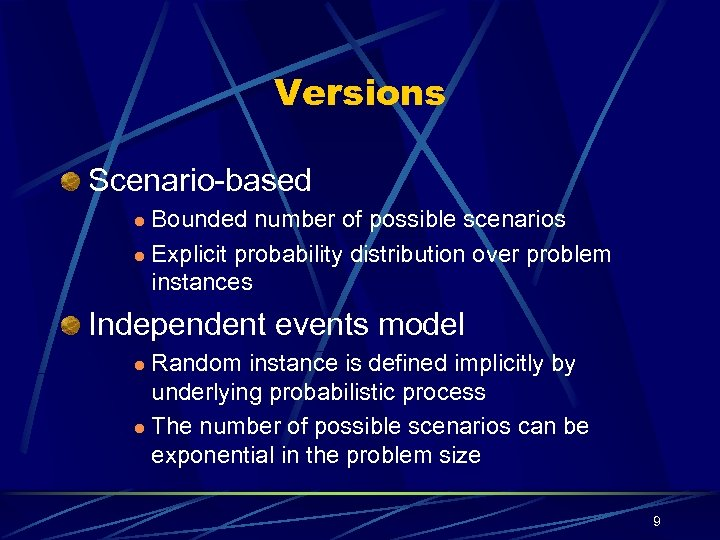Versions Scenario-based Bounded number of possible scenarios l Explicit probability distribution over problem instances l Independent events model Random instance is defined implicitly by underlying probabilistic process l The number of possible scenarios can be exponential in the problem size l 9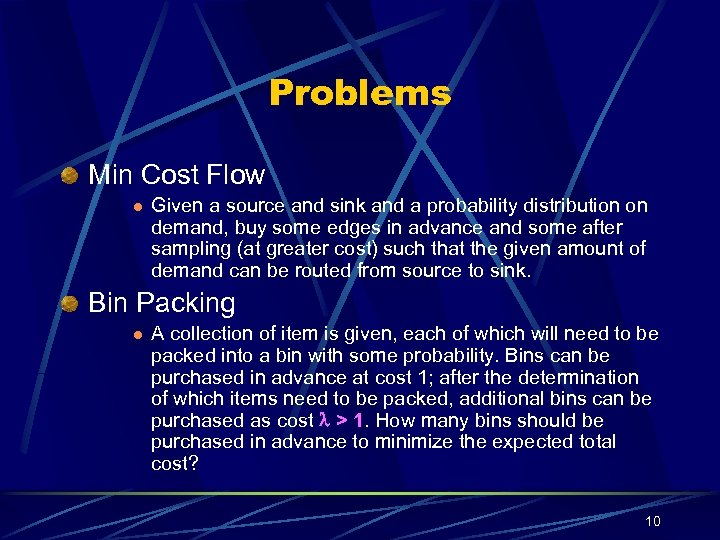Problems Min Cost Flow l Given a source and sink and a probability distribution on demand, buy some edges in advance and some after sampling (at greater cost) such that the given amount of demand can be routed from source to sink. Bin Packing l A collection of item is given, each of which will need to be packed into a bin with some probability. Bins can be purchased in advance at cost 1; after the determination of which items need to be packed, additional bins can be purchased as cost > 1. How many bins should be purchased in advance to minimize the expected total cost? 10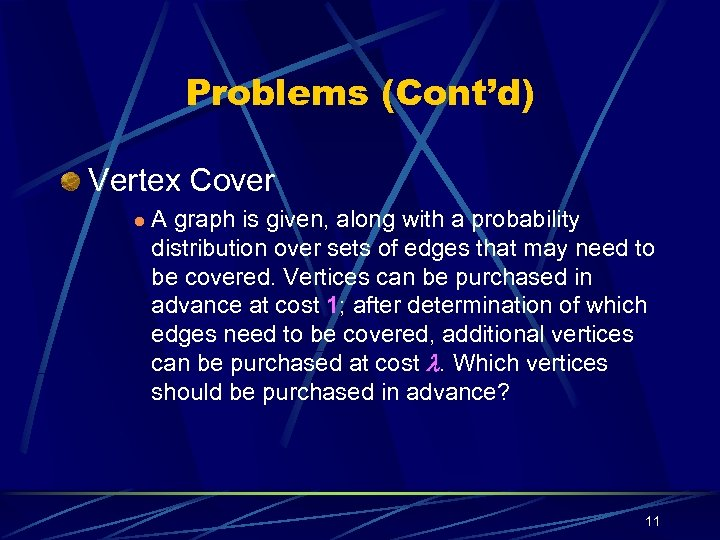Problems (Cont’d) Vertex Cover l A graph is given, along with a probability distribution over sets of edges that may need to be covered. Vertices can be purchased in advance at cost 1; after determination of which edges need to be covered, additional vertices can be purchased at cost . Which vertices should be purchased in advance? 11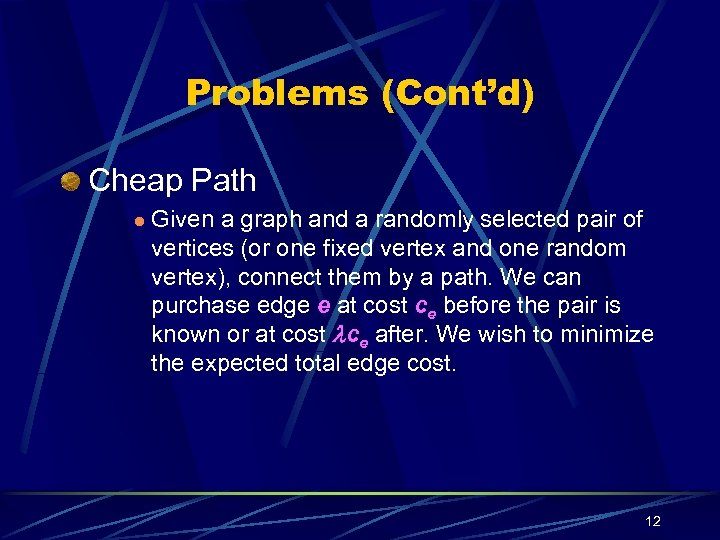Problems (Cont’d) Cheap Path l Given a graph and a randomly selected pair of vertices (or one fixed vertex and one random vertex), connect them by a path. We can purchase edge e at cost ce before the pair is known or at cost ce after. We wish to minimize the expected total edge cost. 12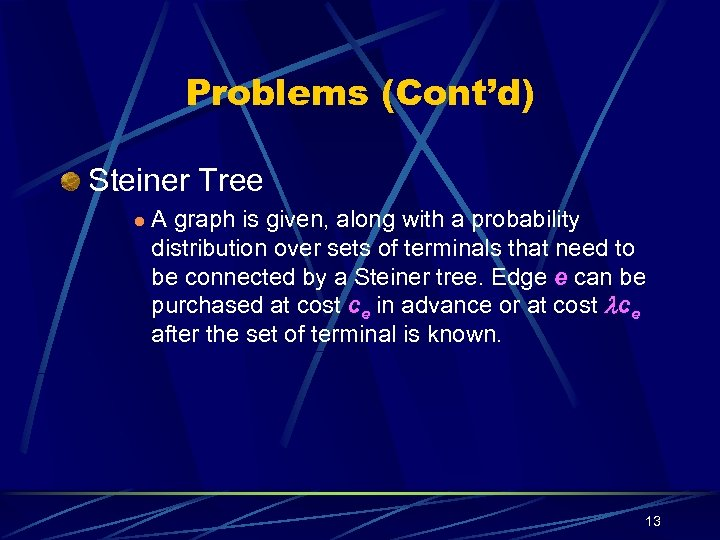Problems (Cont’d) Steiner Tree l A graph is given, along with a probability distribution over sets of terminals that need to be connected by a Steiner tree. Edge e can be purchased at cost ce in advance or at cost ce after the set of terminal is known. 13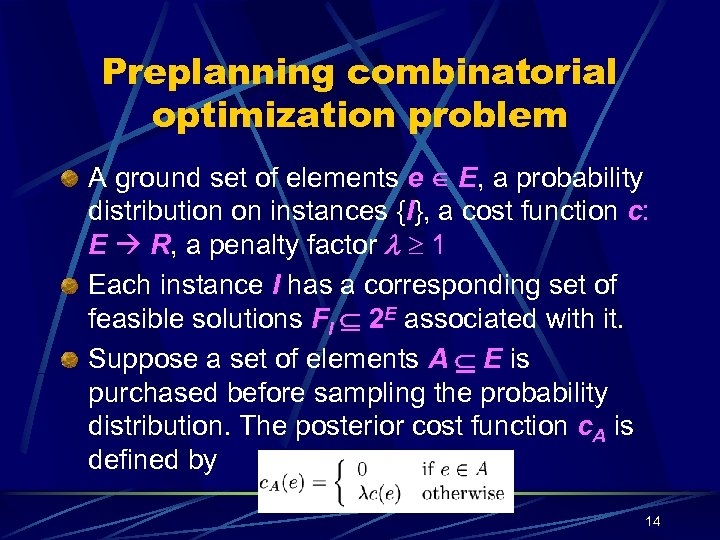Preplanning combinatorial optimization problem A ground set of elements e E, a probability distribution on instances {I}, a cost function c: E R, a penalty factor 1 Each instance I has a corresponding set of feasible solutions FI 2 E associated with it. Suppose a set of elements A E is purchased before sampling the probability distribution. The posterior cost function c. A is defined by 14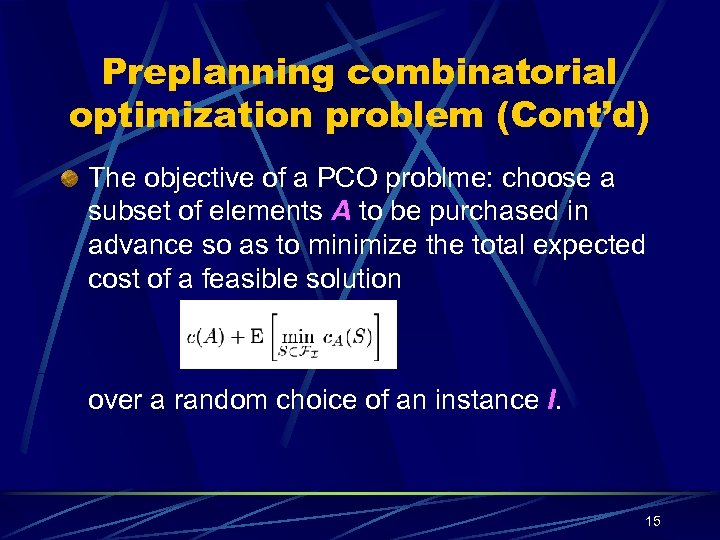Preplanning combinatorial optimization problem (Cont’d) The objective of a PCO problme: choose a subset of elements A to be purchased in advance so as to minimize the total expected cost of a feasible solution over a random choice of an instance I. 15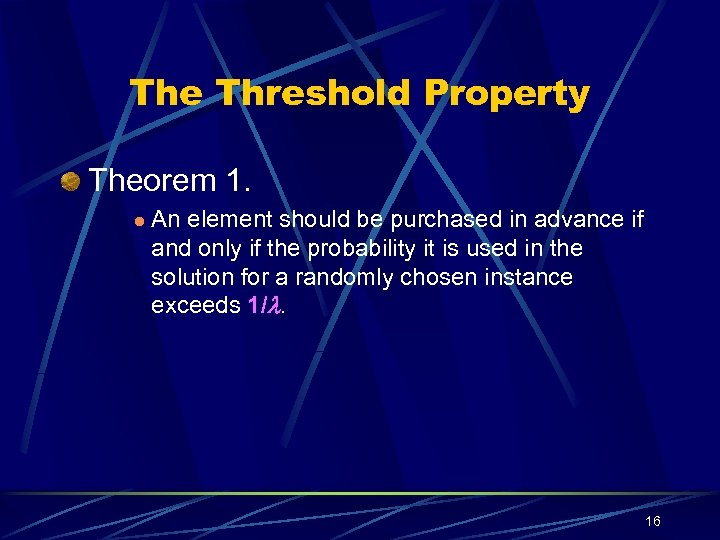The Threshold Property Theorem 1. l An element should be purchased in advance if and only if the probability it is used in the solution for a randomly chosen instance exceeds 1/. 16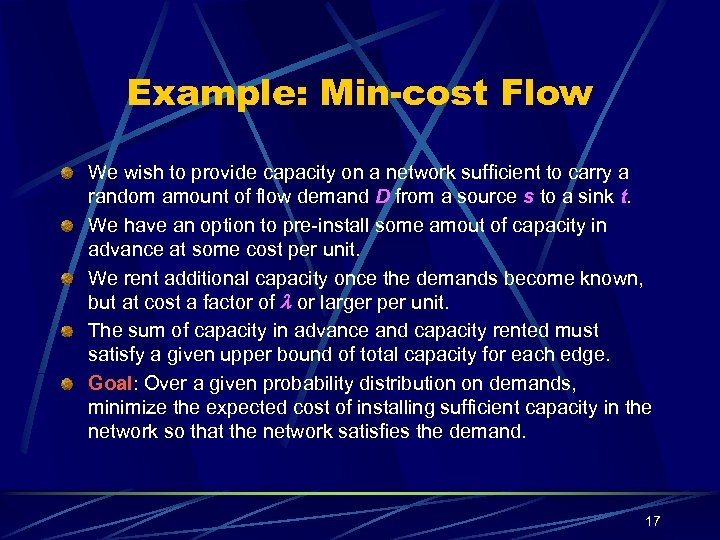Example: Min-cost Flow We wish to provide capacity on a network sufficient to carry a random amount of flow demand D from a source s to a sink t. We have an option to pre-install some amout of capacity in advance at some cost per unit. We rent additional capacity once the demands become known, but at cost a factor of or larger per unit. The sum of capacity in advance and capacity rented must satisfy a given upper bound of total capacity for each edge. Goal: Over a given probability distribution on demands, minimize the expected cost of installing sufficient capacity in the network so that the network satisfies the demand. 17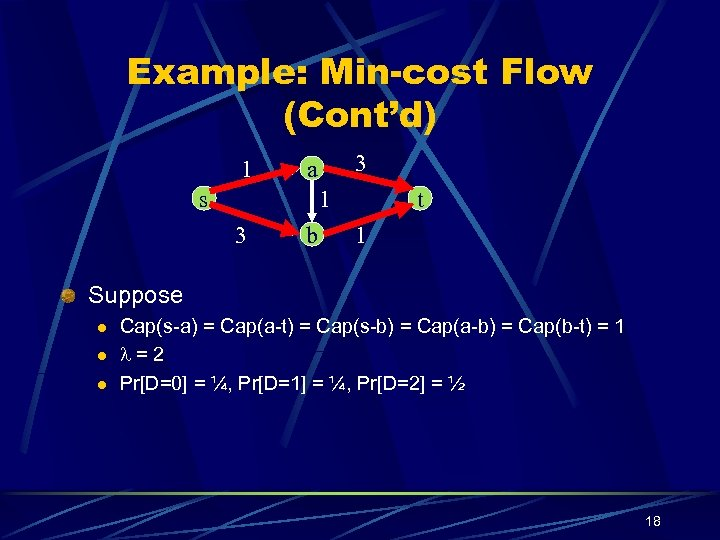Example: Min-cost Flow (Cont’d) 1 3 a s t 1 3 b 1 Suppose l l l Cap(s-a) = Cap(a-t) = Cap(s-b) = Cap(a-b) = Cap(b-t) = 1 =2 Pr[D=0] = ¼, Pr[D=1] = ¼, Pr[D=2] = ½ 18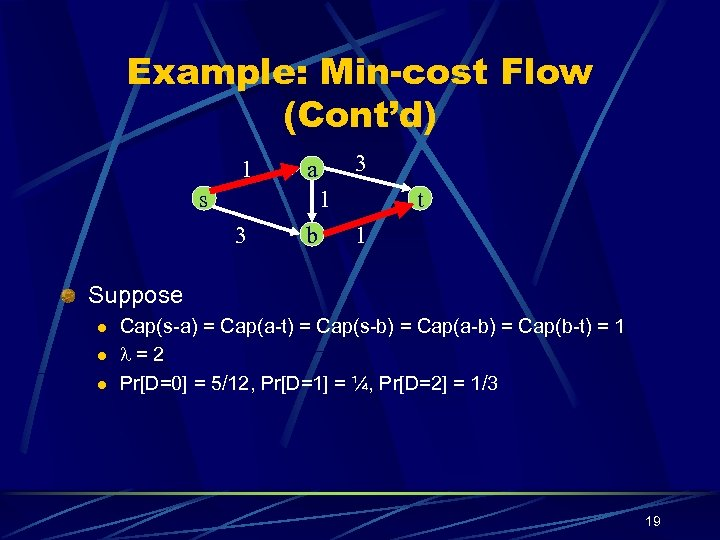Example: Min-cost Flow (Cont’d) 1 3 a s t 1 3 b 1 Suppose l l l Cap(s-a) = Cap(a-t) = Cap(s-b) = Cap(a-b) = Cap(b-t) = 1 =2 Pr[D=0] = 5/12, Pr[D=1] = ¼, Pr[D=2] = 1/3 19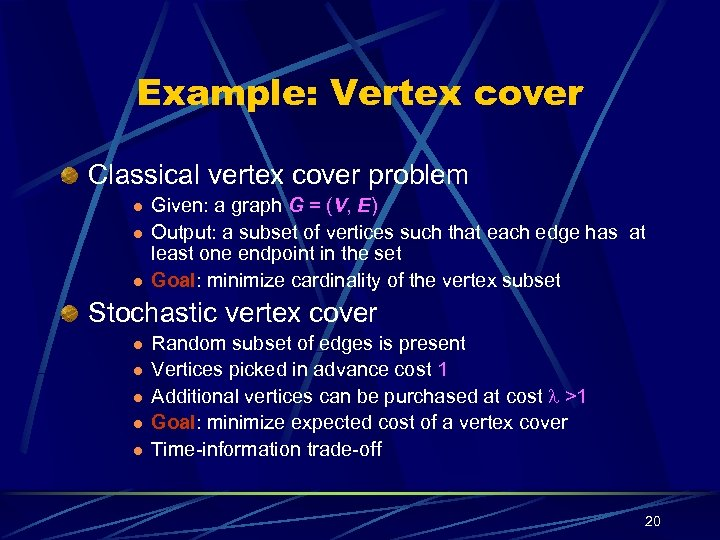Example: Vertex cover Classical vertex cover problem l l l Given: a graph G = (V, E) Output: a subset of vertices such that each edge has at least one endpoint in the set Goal: minimize cardinality of the vertex subset Stochastic vertex cover l l l Random subset of edges is present Vertices picked in advance cost 1 Additional vertices can be purchased at cost >1 Goal: minimize expected cost of a vertex cover Time-information trade-off 20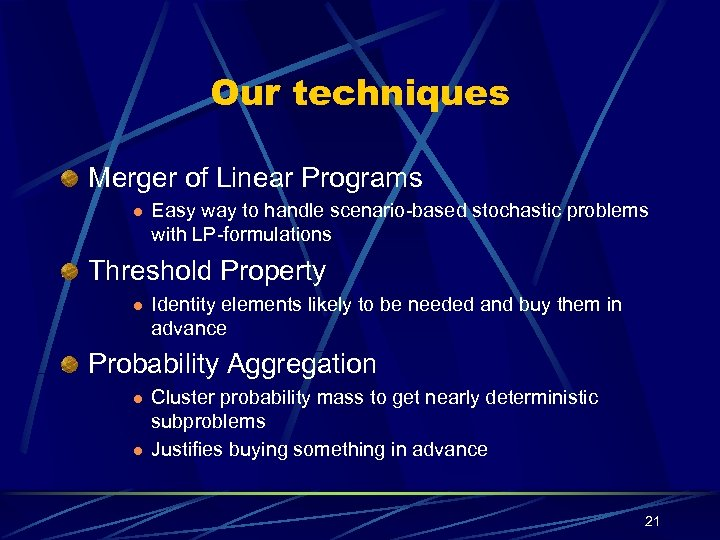Our techniques Merger of Linear Programs l Easy way to handle scenario-based stochastic problems with LP-formulations Threshold Property l Identity elements likely to be needed and buy them in advance Probability Aggregation l l Cluster probability mass to get nearly deterministic subproblems Justifies buying something in advance 21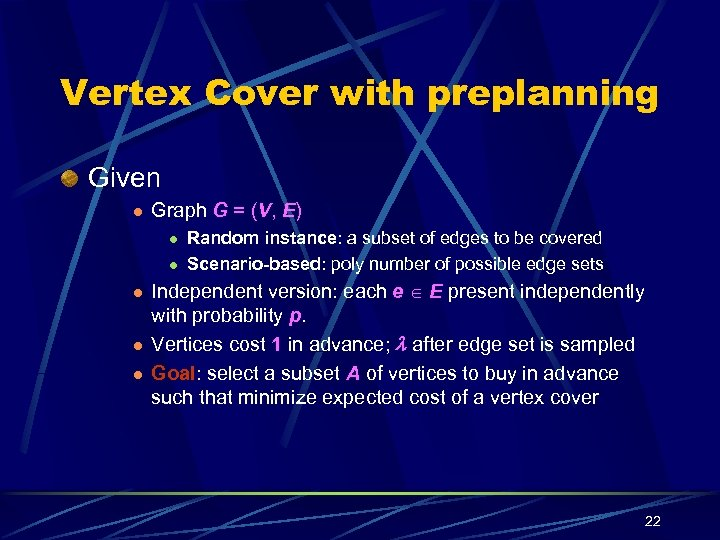Vertex Cover with preplanning Given l Graph G = (V, E) l l l Random instance: a subset of edges to be covered Scenario-based: poly number of possible edge sets Independent version: each e E present independently with probability p. Vertices cost 1 in advance; after edge set is sampled Goal: select a subset A of vertices to buy in advance such that minimize expected cost of a vertex cover 22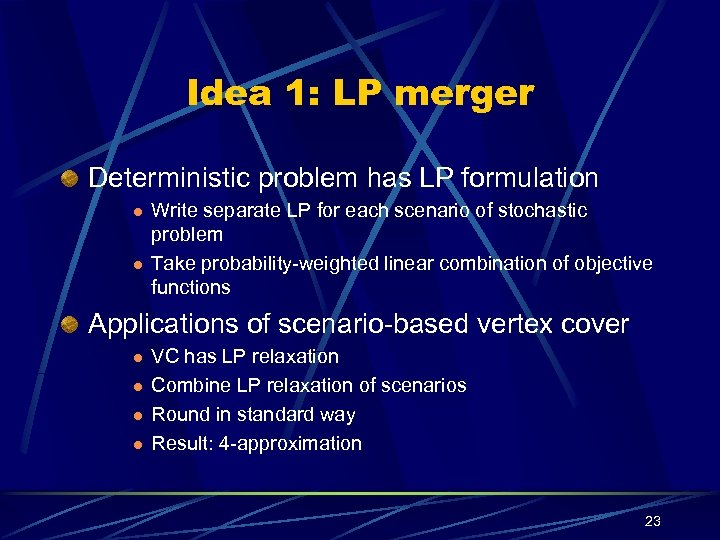Idea 1: LP merger Deterministic problem has LP formulation l l Write separate LP for each scenario of stochastic problem Take probability-weighted linear combination of objective functions Applications of scenario-based vertex cover l l VC has LP relaxation Combine LP relaxation of scenarios Round in standard way Result: 4 -approximation 23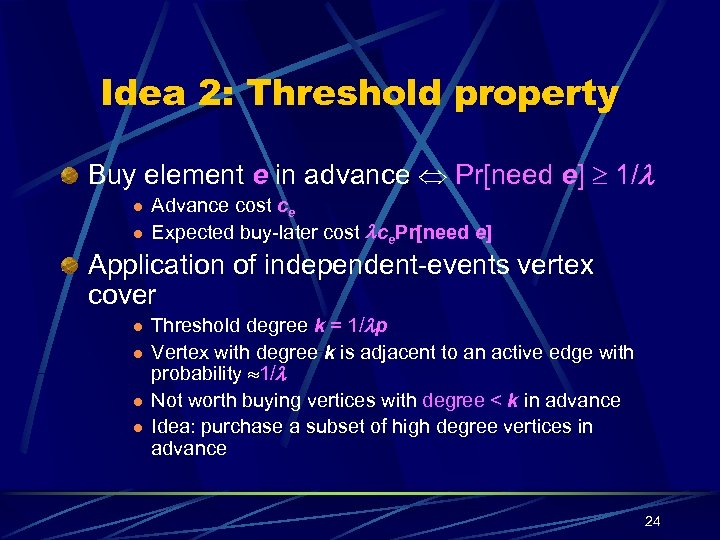Idea 2: Threshold property Buy element e in advance Pr[need e] 1/ l l Advance cost ce Expected buy-later cost ce. Pr[need e] Application of independent-events vertex cover l l Threshold degree k = 1/ p Vertex with degree k is adjacent to an active edge with probability 1/ Not worth buying vertices with degree < k in advance Idea: purchase a subset of high degree vertices in advance 24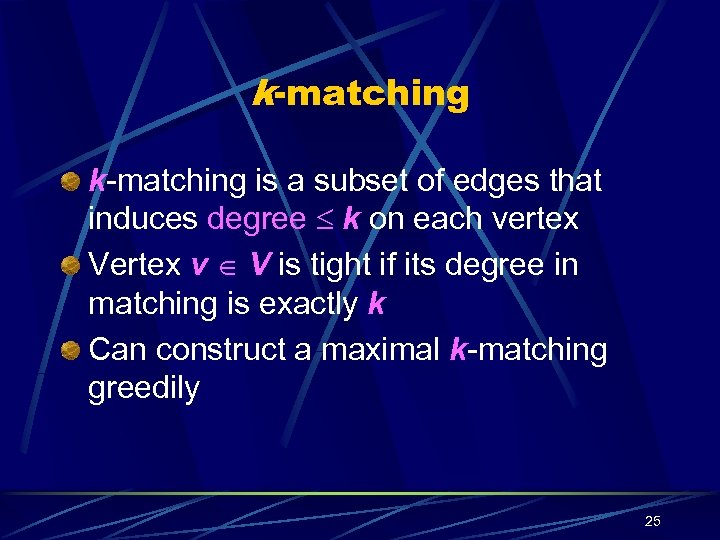k-matching is a subset of edges that induces degree k on each vertex Vertex v V is tight if its degree in matching is exactly k Can construct a maximal k-matching greedily 25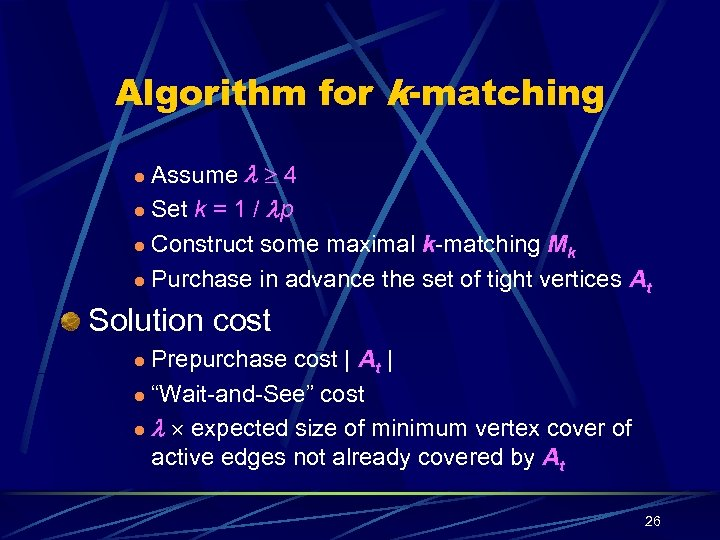Algorithm for k-matching Assume 4 l Set k = 1 / p l Construct some maximal k-matching Mk l Purchase in advance the set of tight vertices At l Solution cost Prepurchase cost | At | l “Wait-and-See” cost l expected size of minimum vertex cover of active edges not already covered by At l 26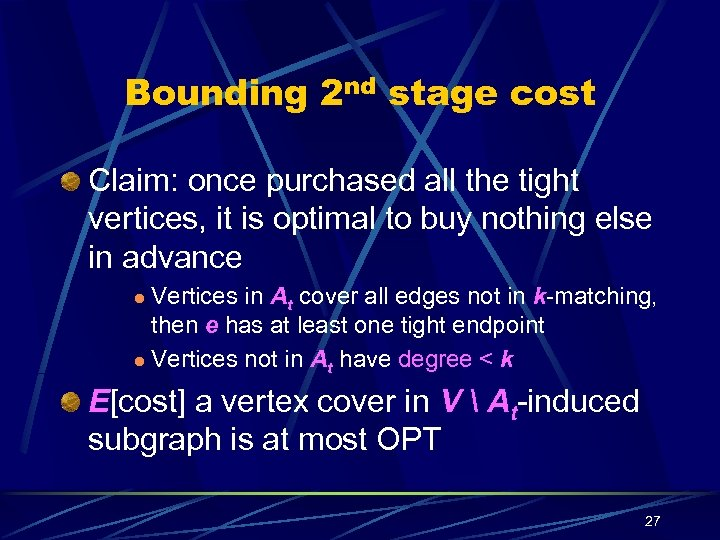Bounding 2 nd stage cost Claim: once purchased all the tight vertices, it is optimal to buy nothing else in advance Vertices in At cover all edges not in k-matching, then e has at least one tight endpoint l Vertices not in At have degree < k l E[cost] a vertex cover in V At-induced subgraph is at most OPT 27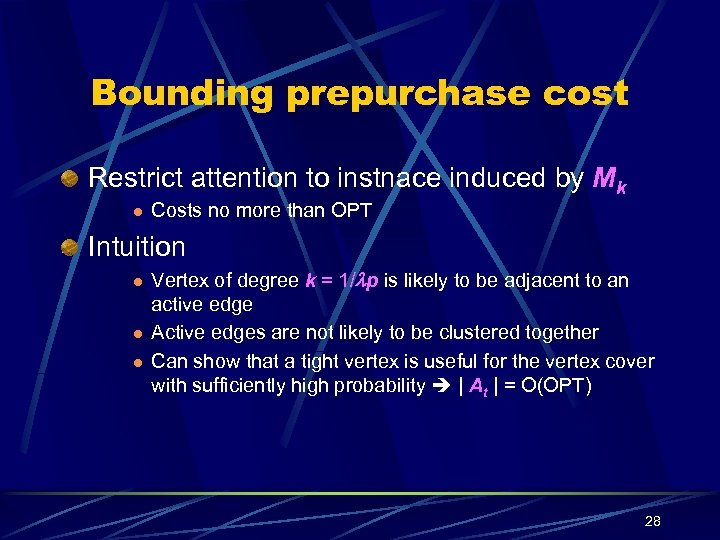Bounding prepurchase cost Restrict attention to instnace induced by Mk l Costs no more than OPT Intuition l l l Vertex of degree k = 1/ p is likely to be adjacent to an active edge Active edges are not likely to be clustered together Can show that a tight vertex is useful for the vertex cover with sufficiently high probability | At | = O(OPT) 28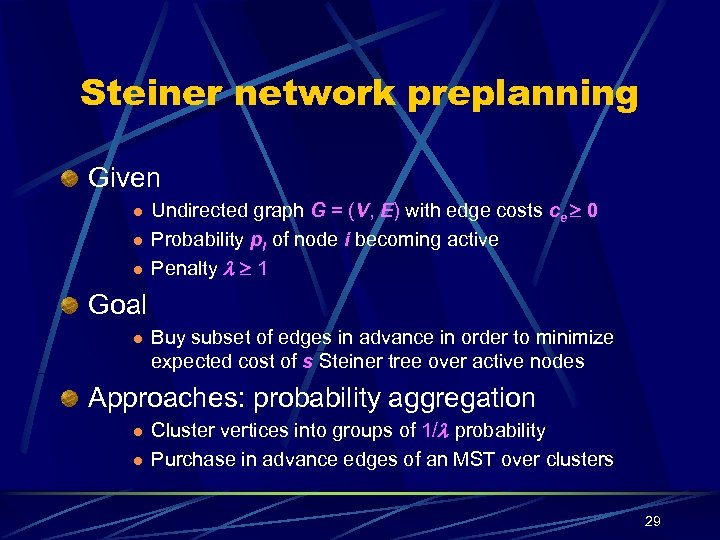Steiner network preplanning Given l l l Undirected graph G = (V, E) with edge costs ce 0 Probability pi of node i becoming active Penalty 1 Goal l Buy subset of edges in advance in order to minimize expected cost of s Steiner tree over active nodes Approaches: probability aggregation l l Cluster vertices into groups of 1/ probability Purchase in advance edges of an MST over clusters 29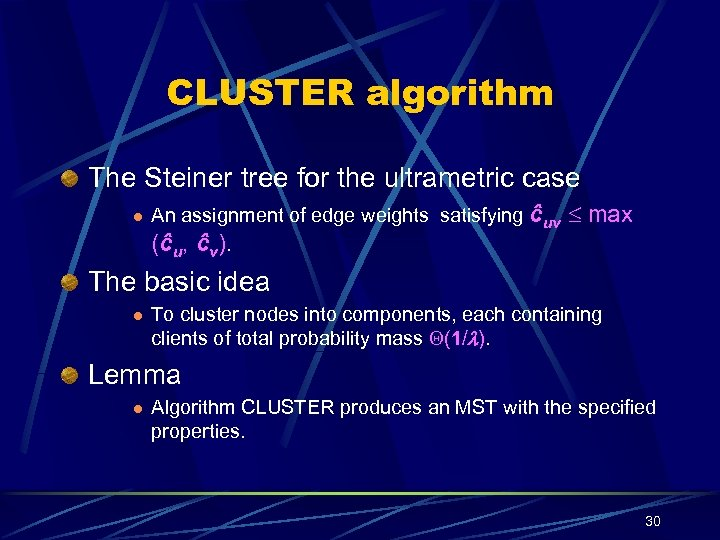CLUSTER algorithm The Steiner tree for the ultrametric case l An assignment of edge weights satisfying ĉuv (ĉu, ĉv). max The basic idea l To cluster nodes into components, each containing clients of total probability mass (1/ ). Lemma l Algorithm CLUSTER produces an MST with the specified properties. 30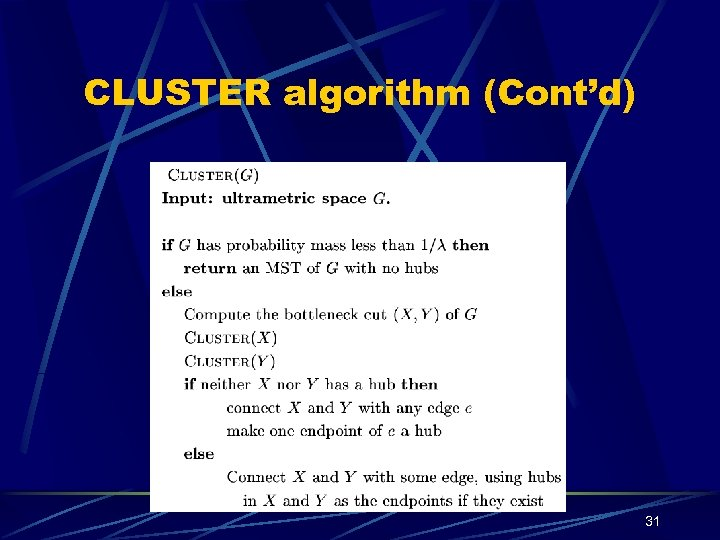CLUSTER algorithm (Cont’d) 31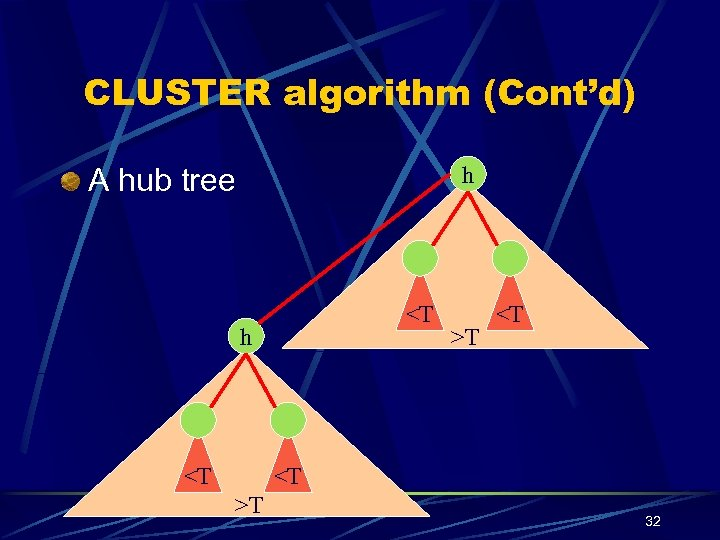CLUSTER algorithm (Cont’d) h A hub tree T T 32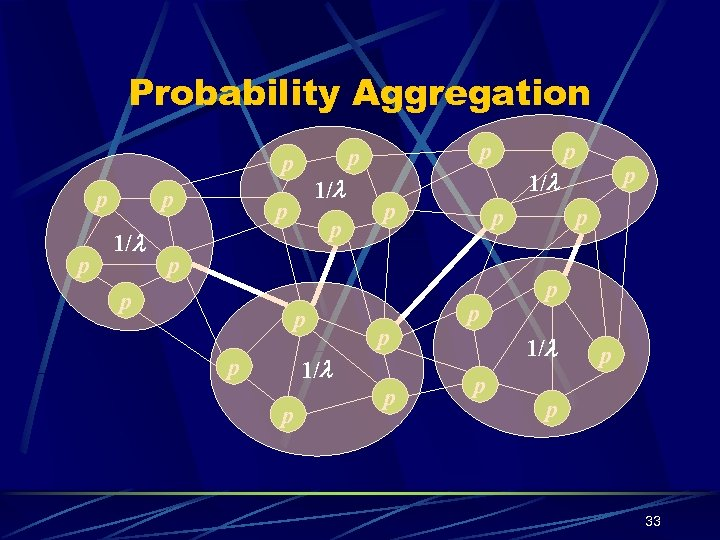Probability Aggregation p p p 1/ p p p 1/ p p p p 1/ p p p 33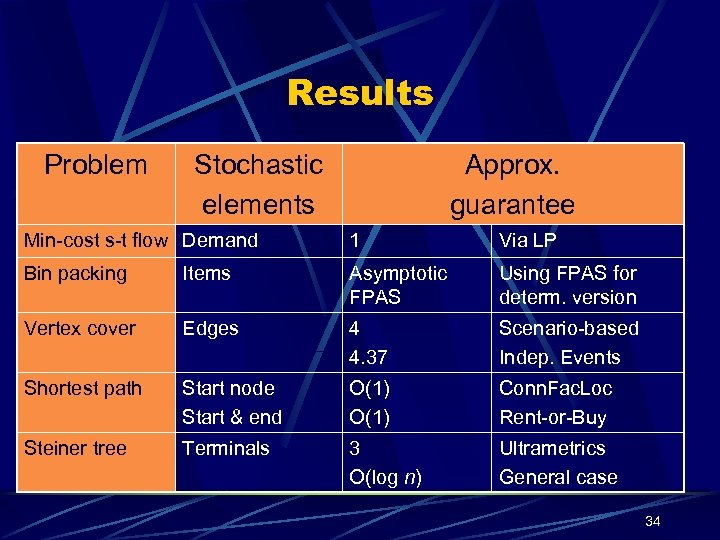Results Problem Stochastic elements Approx. guarantee Min-cost s-t flow Demand 1 Via LP Bin packing Items Asymptotic FPAS Using FPAS for determ. version Vertex cover Edges 4 4. 37 Scenario-based Indep. Events Shortest path Start node Start & end O(1) Conn. Fac. Loc Rent-or-Buy Steiner tree Terminals 3 O(log n) Ultrametrics General case 34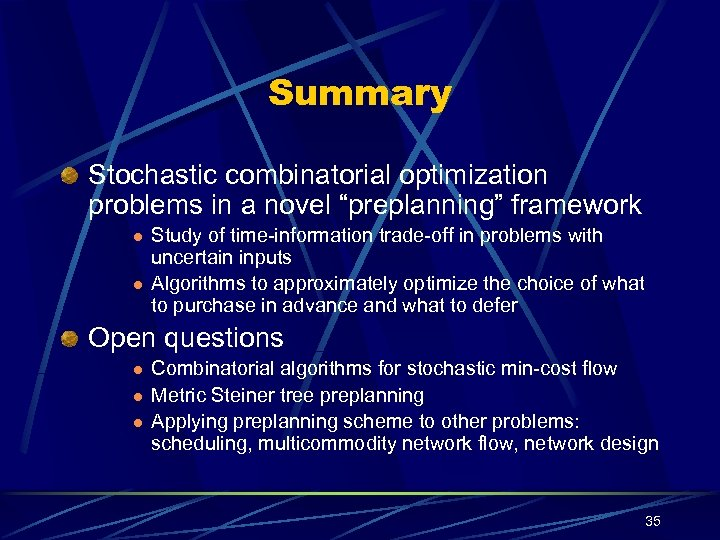Summary Stochastic combinatorial optimization problems in a novel “preplanning” framework l l Study of time-information trade-off in problems with uncertain inputs Algorithms to approximately optimize the choice of what to purchase in advance and what to defer Open questions l l l Combinatorial algorithms for stochastic min-cost flow Metric Steiner tree preplanning Applying preplanning scheme to other problems: scheduling, multicommodity network flow, network design 35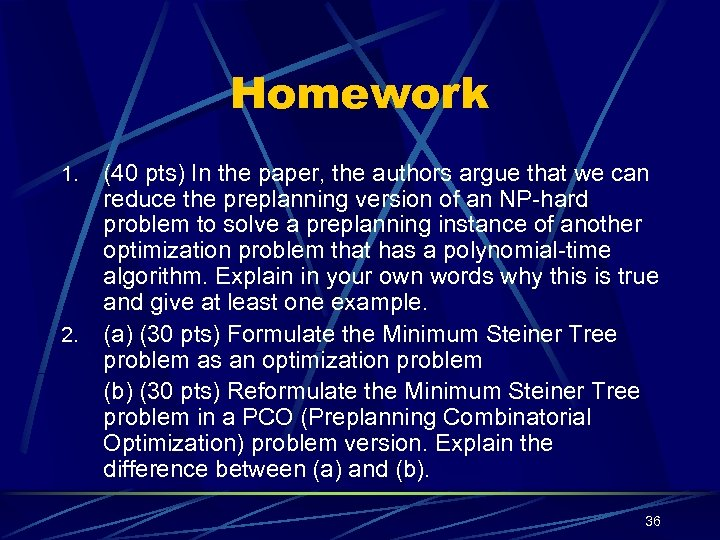Homework 1. 2. (40 pts) In the paper, the authors argue that we can reduce the preplanning version of an NP-hard problem to solve a preplanning instance of another optimization problem that has a polynomial-time algorithm. Explain in your own words why this is true and give at least one example. (a) (30 pts) Formulate the Minimum Steiner Tree problem as an optimization problem (b) (30 pts) Reformulate the Minimum Steiner Tree problem in a PCO (Preplanning Combinatorial Optimization) problem version. Explain the difference between (a) and (b). 36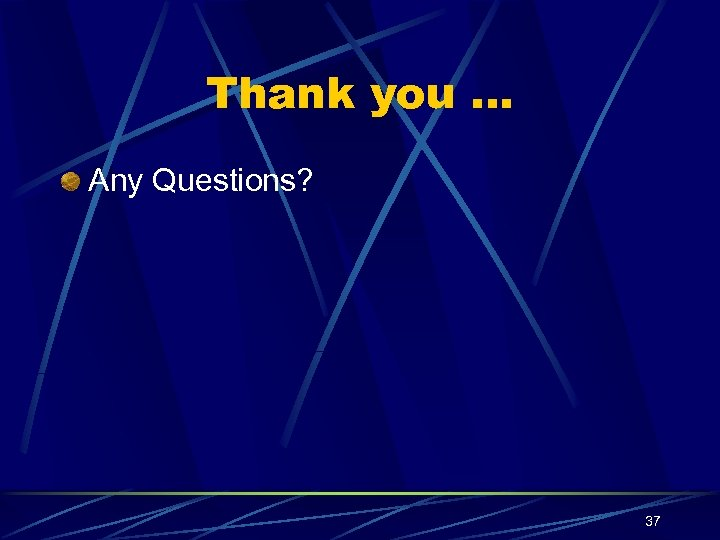Thank you … Any Questions? 37